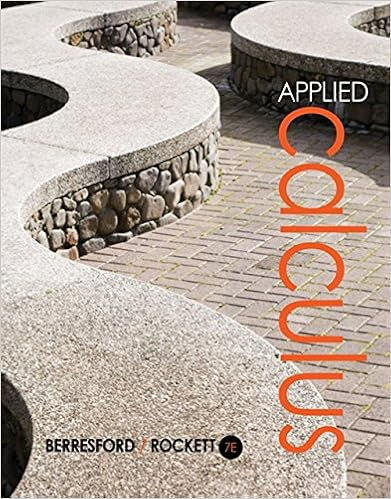# Check the solution x 4 in the original equation 2 7 2

• Test Prep
• 285

This preview shows page 273 - 277 out of 285 pages.

##### We have textbook solutions for you!
The document you are viewing contains questions related to this textbook.The document you are viewing contains questions related to this textbook.
Chapter 2 / Exercise 84
Applied Calculus
Berresford/RockettExpert Verified
Check the solution x= 4 in the original equation.2 72(4) = (4)Simplify the expression.16 = (4) or 4 = 4This is not true. There is one solution for the original equation, x= 12.501 Algebra Questions
##### We have textbook solutions for you!
The document you are viewing contains questions related to this textbook.The document you are viewing contains questions related to this textbook.
Chapter 2 / Exercise 84
Applied Calculus
Berresford/RockettExpert Verified
475.Square both sides of the equation.x2= 32x+ 10Add (23x10) to both sides of the equation.x232x10 = 0Multiply the equation by 2 to eliminate the fraction.2x23x20 = 0Factor using the trinomial factor form.2x23x20 = (x4)(2x+ 5) = 0Letting each factor of the trinomial factors equal zero results in two possible solutions for the original equation, x= 4 and/or x= 212.Check the first possible solution in the original equation.4 = 32(4) + 10Simplify the radical expression.4 = 16 or 4 = 4The solution x= 4 checks out as a solution for the original equation.Check the second possible solution in the original equation.212= 32(212) + 10A negative number cannot be equal to a positive square root as the radical sign in the original expression calls for. Therefore, x= 212is nota solution to the original equation. The only solution for this equation is x= 4.260501 Algebra Questions
•••